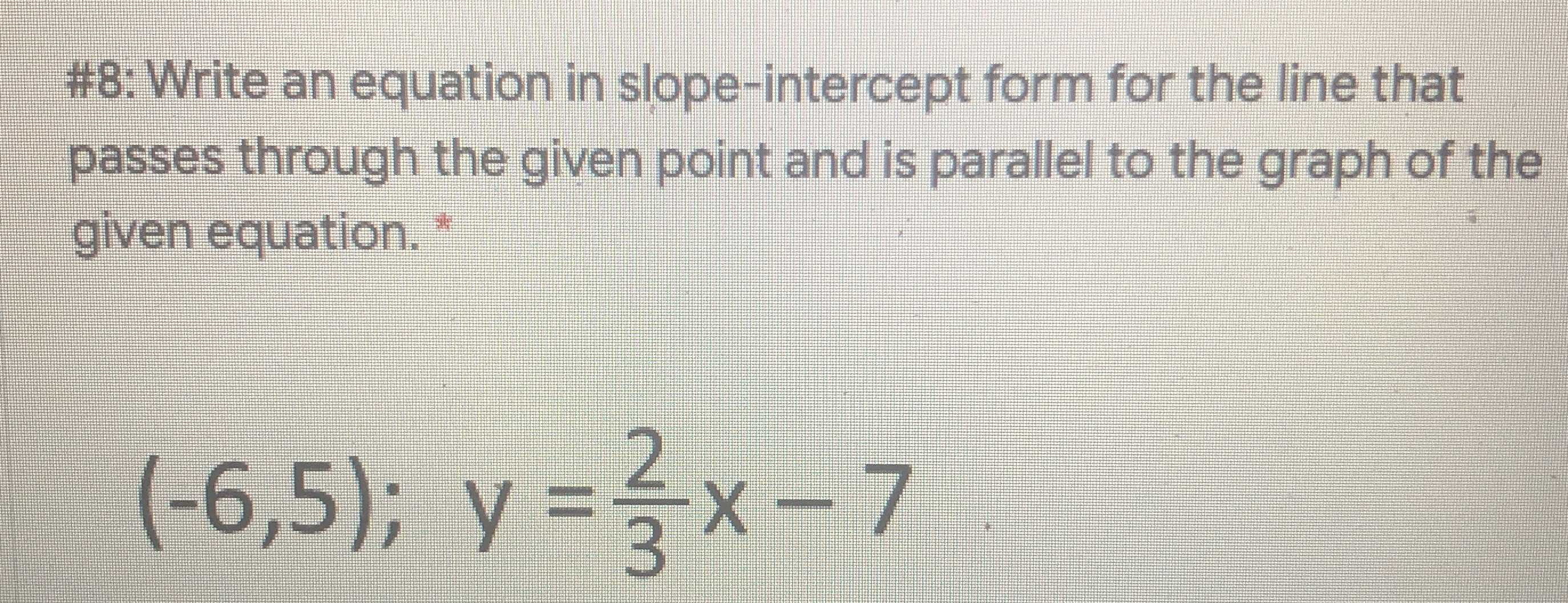### ¿Todavía tienes preguntas de matemáticas?

Pregunte a nuestros tutores expertos
Algebra
Pregunta#8: Write an equation in slope-intercept form for the line that passes through the given point and is parallel to the graph of the given equation. *

$$( - 6,5 ) ; y = \frac { 2 } { 3 } x - 7$$

$$y= \frac{2}{3}x+ 9$$